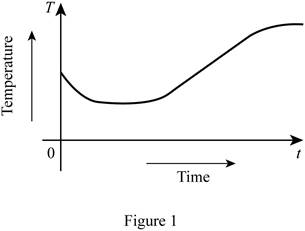# A rough graph of the temperature of the water as a function of the elapsed time.### Single Variable Calculus: Concepts...

4th Edition
James Stewart
Publisher: Cengage Learning
ISBN: 9781337687805### Single Variable Calculus: Concepts...

4th Edition
James Stewart
Publisher: Cengage Learning
ISBN: 9781337687805

#### Solutions

Chapter 1.1, Problem 11E
To determine

## To sketch: A rough graph of the temperature of the water as a function of the elapsed time.

Expert Solution

### Explanation of Solution

Given:

Some ice cubes are put in a glass filled with cold water and then it is placed on the table and cooled.

Observation:

Let the time in x-axis and the temperature in y-axis. Initially when ice cubes are put in a glass filled with cold water, the temperature of water is freeze as ice melts. And then the temperature increased and reaches the room temperature as the ice melted. Thus, the rough graph of the temperature of the pie as a function of time is shown below in Figure 1.From Figure 1, it is observed that the temperature is decreasing at the beginning, remains constant in the middle and then increasing and reaches the room temperature.

### Have a homework question?

Subscribe to bartleby learn! Ask subject matter experts 30 homework questions each month. Plus, you’ll have access to millions of step-by-step textbook answers!